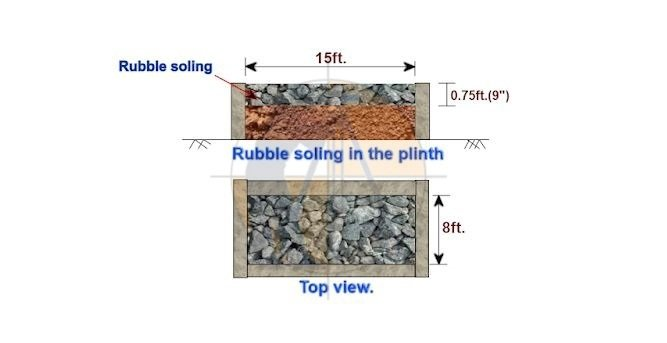Breaking News
Home / Civil Engineering / Estimation And Rate Analysis of Rubble Soling Work

# Estimation And Rate Analysis of Rubble Soling Work

## Estimation And Rate Analysis of Rubble Soling Work

Let us now calculate the cost of Rubble soling as shown in the below drawing.Given data:

Length of the rubble soling = 15 ft.

Width of the rubble soling = 8 ft.

The thickness of the rubble soling = 0.75 ft.

The total volume of the rubble soling

= [length × width × thickness]

= [15 ft. × 8 ft. × 0.75 ft.]

90 cu ft.

Before proceeding further, go through the article.

### Unit Weight of Different Building Materials

So that you will understand the calculation concept clearly, without any questions.

1. Material calculation:
2. Volume of  rubble stone:

The required volume of stone

= [1.25 × the vol. of  rubble soling]

= [1.25 × 90 cu ft.]

112.50 cu ft.

1. Volume of moo-rum:

The required vol. of moo-rum

= 15% of the vol. of rubble stone.

= [15 × 112.5 cu ft. ÷ 100]

= 16.875 cu ft.

1. Labor calculation:

The labor rate per cu ft. of the rubble soling work ranges from INR 9/- to 15/- depending upon the region.

Let us consider an average rate of INR 12/– per cu ft.

The total cost of labor for rubble soling

= total vol. of rubble soling in cu ft.  × labor rate/ cu ft.

= 90 cu ft. × 12/-

INR 1,080/-

### Building Estimation of Long Wall Short Wall And Center Line Method.

Note: Labor rate inclusive of moo-rum spreading, compaction, and water flooding.

Now, let us calculate the total cost of rubble soling in table format.

 Sl. no. Item. Qty. Rate. in INR. Unit. Cost in INR. 1. Rubble stone 112.50 33/- Cu ft. 3,712.50 2. Moorum 16.88 12/- Cu ft. 202.56 3. Rent of compactor Lump-sum 200.00 4. Water for flooding Lump-sum 100.00 5. Miscellaneous Lump-sum 100.00 6. The material cost = 4,315.06 7. Add 5% wastage = 215.75 8. Total material cost = 4,530.81 9. Labour cost = 1,080.00 10. The total cost of rubble soling work = 5610.81

Note: To get accurate results, insert your regional market rate of materials and labor, in the above table.

1. The cost of rubble soling /cu ft.

= [total rubble soling cost ÷ total soling vol. in cu ft.]

= [5,610.81 ÷ 90]

= INR 62.34 /cu ft.

### Load Calculation On Column, Beam, Wall And Slab

1. The cost of rubble soling /cum.

= [62.34 × 35.3147]

= INR 2,201.60 / cum.

( As 1cum = 35.3147 cu ft.)

Note:

If the work is handed over to any contractor, you have to add 10% extra for his profit.

## . Land Surveying & Architects

### THANKS.